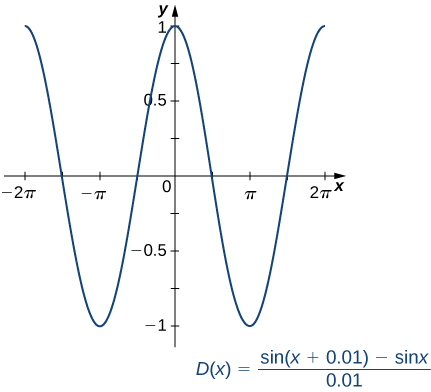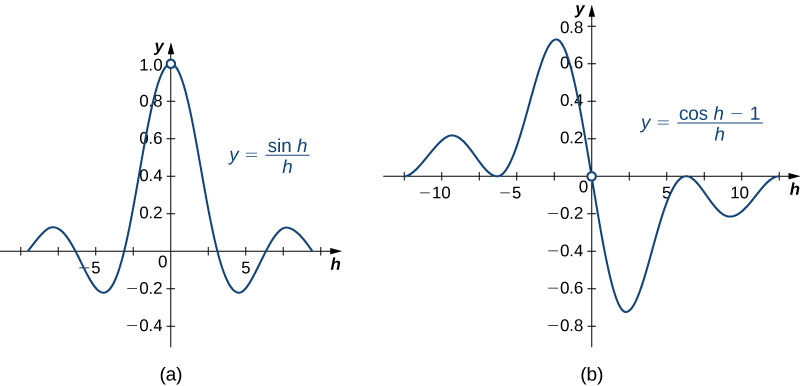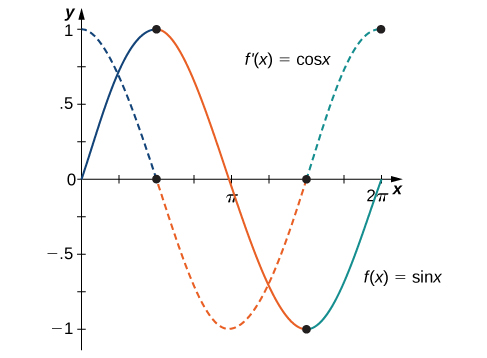## 3.5 Derivatives of Trigonometric Functions

### Learning Objectives

• Find the derivatives of the sine and cosine function.
• Find the derivatives of the standard trigonometric functions.
• Calculate the higher-order derivatives of the sine and cosine.

One of the most important types of motion in physics is simple harmonic motion, which is associated with such systems as an object with mass oscillating on a spring. Simple harmonic motion can be described by using either sine or cosine functions. In this section we expand our knowledge of derivative formulas to include derivatives of these and other trigonometric functions. We begin with the derivatives of the sine and cosine functions and then use them to obtain formulas for the derivatives of the remaining four trigonometric functions. Being able to calculate the derivatives of the sine and cosine functions will enable us to find the velocity and acceleration of simple harmonic motion.

# Derivatives of the Sine and Cosine Functions

We begin our exploration of the derivative for the sine function by using the formula to make a reasonable guess at its derivative. Recall that for a function $f(x),$

$f^{\prime}(x)=\underset{h\to 0}{\lim}\frac{f(x+h)-f(x)}{h}$.

Consequently, for values of $h$ very close to 0, $f^{\prime}(x)\approx \frac{f(x+h)-f(x)}{h}$. We see that by using $h=0.01$,

$\frac{d}{dx}(\sin x)\approx \frac{\sin(x+0.01)-\sin x}{0.01}$

By setting $D(x)=\frac{\sin(x+0.01)-\sin x}{0.01}$ and using a graphing utility, we can get a graph of an approximation to the derivative of $\sin x$ ((Figure)).Figure 1. The graph of the function $D(x)$ looks a lot like a cosine curve.

Upon inspection, the graph of $D(x)$ appears to be very close to the graph of the cosine function. Indeed, we will show that

$\frac{d}{dx}(\sin x)= \cos x$.

If we were to follow the same steps to approximate the derivative of the cosine function, we would find that

$\frac{d}{dx}(\cos x)=−\sin x$.

### The Derivatives of $\sin x$ and $\cos x$

The derivative of the sine function is the cosine and the derivative of the cosine function is the negative sine.

$\frac{d}{dx}(\sin x)= \cos x$
$\frac{d}{dx}(\cos x)=−\sin x$

## Proof

Because the proofs for $\frac{d}{dx}(\sin x)= \cos x$ and $\frac{d}{dx}(\cos x)=−\sin x$ use similar techniques, we provide only the proof for $\frac{d}{dx}(\sin x)= \cos x$. Before beginning, recall two important trigonometric limits we learned in Introduction to Limits:

$\underset{h\to 0}{\lim}\frac{\sin h}{h}=1$ and $\underset{h\to 0}{\lim}\frac{\cos h-1}{h}=0$.

The graphs of $y=\frac{\sin h}{h}$ and $y=\frac{(\cos h-1)}{h}$ are shown in (Figure).Figure 2. These graphs show two important limits needed to establish the derivative formulas for the sine and cosine functions.

We also recall the following trigonometric identity for the sine of the sum of two angles:

$\sin(x+h)= \sin x \cos h+ \cos x \sin h$.

Now that we have gathered all the necessary equations and identities, we proceed with the proof.

$\begin{array}{lllll}\frac{d}{dx} \sin x & =\underset{h\to 0}{\lim}\frac{\sin(x+h)-\sin x}{h} & & & \text{Apply the definition of the derivative.} \\ & =\underset{h\to 0}{\lim}\frac{\sin x \cos h+ \cos x \sin h- \sin x}{h} & & & \text{Use trig identity for the sine of the sum of two angles.} \\ & =\underset{h\to 0}{\lim}(\frac{\sin x \cos h-\sin x}{h}+\frac{\cos x \sin h}{h}) & & & \text{Regroup.} \\ & =\underset{h\to 0}{\lim}(\sin x(\frac{\cos h-1}{h})+ \cos x(\frac{\sin h}{h})) & & & \text{Factor out} \, \sin x \, \text{and} \, \cos x. \\ & = \sin x(0)+ \cos x(1) & & & \text{Apply trig limit formulas.} \\ & = \cos x & & & \text{Simplify.} \end{array} _\blacksquare$

(Figure) shows the relationship between the graph of $f(x)= \sin x$ and its derivative $f^{\prime}(x)= \cos x$. Notice that at the points where $f(x)= \sin x$ has a horizontal tangent, its derivative $f^{\prime}(x)= \cos x$ takes on the value zero. We also see that where $f(x)= \sin x$ is increasing, $f^{\prime}(x)= \cos x>0$ and where $f(x)= \sin x$ is decreasing, $f^{\prime}(x)= \cos x<0$.Figure 3. Where $f(x)$ has a maximum or a minimum, $f^{\prime}(x)=0$. That is, $f^{\prime}(x)=0$ where $f(x)$ has a horizontal tangent. These points are noted with dots on the graphs.

### Differentiating a Function Containing $\sin x$

Find the derivative of $f(x)=5x^3 \sin x$.

Find the derivative of $f(x)= \sin x \cos x.$

#### Hint

Don’t forget to use the product rule.

### Finding the Derivative of a Function Containing $\cos x$

Find the derivative of $g(x)=\frac{\cos x}{4x^2}$.

Find the derivative of $f(x)=\frac{x}{\cos x}$.

#### Hint

Use the quotient rule.

### An Application to Velocity

A particle moves along a coordinate axis in such a way that its position at time $t$ is given by $s(t)=2 \sin t-t$ for $0\le t\le 2\pi$. At what times is the particle at rest?

A particle moves along a coordinate axis. Its position at time $t$ is given by $s(t)=\sqrt{3}t+2 \cos t$ for $0\le t\le 2\pi$. At what times is the particle at rest?

#### Hint

Use the previous example as a guide.

# Derivatives of Other Trigonometric Functions

Since the remaining four trigonometric functions may be expressed as quotients involving sine, cosine, or both, we can use the quotient rule to find formulas for their derivatives.

### The Derivative of the Tangent Function

Find the derivative of $f(x)= \tan x$.

Find the derivative of $f(x)= \cot x$.

#### Hint

Rewrite $\cot x$ as $\frac{\cos x}{\sin x}$ and use the quotient rule.

The derivatives of the remaining trigonometric functions may be obtained by using similar techniques. We provide these formulas in the following theorem.

### Derivatives of $\tan x, \, \cot x, \, \sec x$, and $\csc x$

The derivatives of the remaining trigonometric functions are as follows:

$\frac{d}{dx}(\tan x)=\sec^2 x$
$\frac{d}{dx}(\cot x)=−\csc^2 x$
$\frac{d}{dx}(\sec x)= \sec x \tan x$
$\frac{d}{dx}(\csc x)=−\csc x \cot x$

### Finding the Equation of a Tangent Line

Find the equation of a line tangent to the graph of $f(x)= \cot x$ at $x=\frac{\pi}{4}$.

### Finding the Derivative of Trigonometric Functions

Find the derivative of $f(x)= \csc x+x \tan x.$

Find the derivative of $f(x)=2 \tan x-3 \cot x$.

#### Hint

Use the rule for differentiating a constant multiple and the rule for differentiating a difference of two functions.

Find the slope of the line tangent to the graph of $f(x)= \tan x$ at $x=\frac{\pi}{6}$.

#### Hint

Evaluate the derivative at $x=\frac{\pi}{6}$.

# Higher-Order Derivatives

The higher-order derivatives of $\sin x$ and $\cos x$ follow a repeating pattern. By following the pattern, we can find any higher-order derivative of $\sin x$ and $\cos x$.

### Finding Higher-Order Derivatives of $y= \sin x$

Find the first four derivatives of $y= \sin x$.

#### Analysis

Once we recognize the pattern of derivatives, we can find any higher-order derivative by determining the step in the pattern to which it corresponds. For example, every fourth derivative of $\sin x$ equals $\sin x$, so

$\begin{array}{l}\frac{d^4}{dx^4}(\sin x)=\frac{d^8}{dx^8}(\sin x)=\frac{d^{12}}{dx^{12}}(\sin x)=\cdots =\frac{d^{4n}}{dx^{4n}}(\sin x)= \sin x \\ \frac{d^5}{dx^5}(\sin x)=\frac{d^9}{dx^9}(\sin x)=\frac{d^{13}}{dx^{13}}(\sin x)=\cdots =\frac{d^{4n+1}}{dx^{4n+1}}(\sin x)= \cos x\end{array}$

For $y= \cos x$, find $\frac{d^4 y}{dx^4}$.

#### Hint

See the previous example.

### Using the Pattern for Higher-Order Derivatives of $y= \sin x$

Find $\frac{d^{74}}{dx^{74}}(\sin x)$.

For $y= \sin x$, find $\frac{d^{59}}{dx^{59}}(\sin x)$.

#### Hint

$\frac{d^{59}}{dx^{59}}(\sin x)=\frac{d^{4(14)+3}}{dx^{4(14)+3}}(\sin x)$

### An Application to Acceleration

A particle moves along a coordinate axis in such a way that its position at time $t$ is given by $s(t)=2- \sin t$. Find $v(\pi/4)$ and $a(\pi/4)$. Compare these values and decide whether the particle is speeding up or slowing down.

A block attached to a spring is moving vertically. Its position at time $t$ is given by $s(t)=2 \sin t$. Find $v(\frac{5\pi}{6})$ and $a(\frac{5\pi}{6})$. Compare these values and decide whether the block is speeding up or slowing down.

#### Hint

Use (Figure) as a guide.

### Key Concepts

• We can find the derivatives of $\sin x$ and $\cos x$ by using the definition of derivative and the limit formulas found earlier. The results are
$\frac{d}{dx} \sin x= \cos x$ and $\frac{d}{dx} \cos x=−\sin x$.
• With these two formulas, we can determine the derivatives of all six basic trigonometric functions.

# Key Equations

• Derivative of sine function
$\frac{d}{dx}(\sin x)= \cos x$
• Derivative of cosine function
$\frac{d}{dx}(\cos x)=−\sin x$
• Derivative of tangent function
$\frac{d}{dx}(\tan x)=\sec^2 x$
• Derivative of cotangent function
$\frac{d}{dx}(\cot x)=−\csc^2 x$
• Derivative of secant function
$\frac{d}{dx}(\sec x)= \sec x \tan x$
• Derivative of cosecant function
$\frac{d}{dx}(\csc x)=−\csc x \cot x$

For the following exercises, find $\frac{dy}{dx}$ for the given functions.

1. $y=x^2- \sec x+1$

2. $y=3 \csc x+\frac{5}{x}$

3. $y=x^2 \cot x$

4. $y=x-x^3 \sin x$

5. $y=\frac{\sec x}{x}$

6. $y= \sin x \tan x$

7. $y=(x+ \cos x)(1- \sin x)$

8. $y=\frac{\tan x}{1- \sec x}$

9. $y=\frac{1- \cot x}{1+ \cot x}$

10. $y= \cos x(1+ \csc x)$

For the following exercises, find the equation of the tangent line to each of the given functions at the indicated values of $x$. Then use a calculator to graph both the function and the tangent line to ensure the equation for the tangent line is correct.

11. [T] $f(x)=−\sin x, \, x=0$

12. [T] $f(x)= \csc x, \, x=\frac{\pi}{2}$

13. [T] $f(x)=1+ \cos x, \, x=\frac{3\pi}{2}$

14. [T] $f(x)= \sec x, \, x=\frac{\pi}{4}$

15. [T] $f(x)=x^2- \tan x, \, x=0$

16. [T] $f(x)=5 \cot x, \, x=\frac{\pi}{4}$

For the following exercises, find $\frac{d^2 y}{dx^2}$ for the given functions.

17. $y=x \sin x- \cos x$

18. $y= \sin x \cos x$

19. $y=x-\frac{1}{2} \sin x$

20. $y=\frac{1}{x}+ \tan x$

21. $y=2 \csc x$

22. $y=\sec^2 x$

23. Find all $x$ values on the graph of $f(x)=-3 \sin x \cos x$ where the tangent line is horizontal.

24. Find all $x$ values on the graph of $f(x)=x-2 \cos x$ for $0<x<2\pi$ where the tangent line has a slope of 2.

25. Let $f(x)= \cot x$. Determine the point(s) on the graph of $f$ for $0<x<2\pi$ where the tangent line is parallel to the line $y=-2x$.

26. [T] A mass on a spring bounces up and down in simple harmonic motion, modeled by the function $s(t)=-6 \cos t$ where $s$ is measured in inches and $t$ is measured in seconds. Find the rate at which the spring is oscillating at $t=5$ s.

27. Let the position of a swinging pendulum in simple harmonic motion be given by $s(t)=a \cos t+b \sin t$. Find the constants $a$ and $b$ such that when the velocity is 3 cm/s, $s=0$ and $t=0$.

28. After a diver jumps off a diving board, the edge of the board oscillates with position given by $s(t)=-5 \cos t$ cm at $t$ seconds after the jump.

1. Sketch one period of the position function for $t\ge 0$.
2. Find the velocity function.
3. Sketch one period of the velocity function for $t\ge 0$.
4. Determine the times when the velocity is 0 over one period.
5. Find the acceleration function.
6. Sketch one period of the acceleration function for $t\ge 0$.

29. The number of hamburgers sold at a fast-food restaurant in Pasadena, California, is given by $y=10+5 \sin x$ where $y$ is the number of hamburgers sold and $x$ represents the number of hours after the restaurant opened at 11 a.m. until 11 p.m., when the store closes. Find $y^{\prime}$ and determine the intervals where the number of burgers being sold is increasing.

30. [T] The amount of rainfall per month in Phoenix, Arizona, can be approximated by $y(t)=0.5+0.3 \cos t$, where $t$ is the number of months since January. Find $y^{\prime}$ and use a calculator to determine the intervals where the amount of rain falling is decreasing.

For the following exercises, use the quotient rule to derive the given equations.

31. $\frac{d}{dx}(\cot x)=−\csc^2 x$

32. $\frac{d}{dx}(\sec x)= \sec x \tan x$

33. $\frac{d}{dx}(\csc x)=−\csc x \cot x$

34. Use the definition of derivative and the identity

$\cos (x+h)= \cos x \cos h- \sin x \sin h$ to prove that $\frac{d}{dx}(\cos x)=−\sin x$.

For the following exercises, find the requested higher-order derivative for the given functions.

35. $\frac{d^3 y}{dx^3}$ of $y=3 \cos x$

36. $\frac{d^2 y}{dx^2}$ of $y=3 \sin x+x^2 \cos x$

37. $\frac{d^4 y}{dx^4}$ of $y=5 \cos x$

38. $\frac{d^2 y}{dx^2}$ of $y= \sec x+ \cot x$

39. $\frac{d^3 y}{dx^3}$ of $y=x^{10}- \sec x$